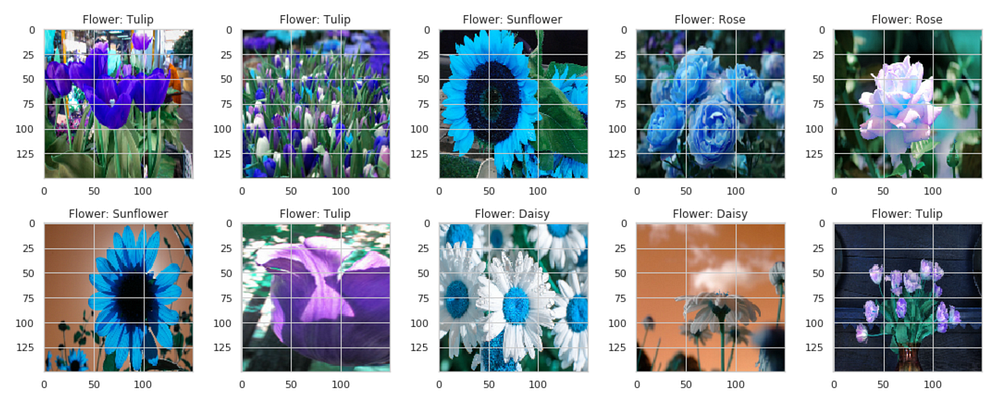# Roses are red, violets are blue, AI can recognize flowers for you

Source: Deep Learning on MediumThis was achieved with Keras’s ImageDataGenerator, which augments the image among some of the following parameters (not exhaustive):

• Rotation — how much the image can be rotated, in degrees.
• Width Shift — how much the image can be shifted left or right.
• Height Shift — how much the image can be shifted up or down.
• Zoom — how much the image can be zoomed.
• Horizontal Flip — if the image can be flipped horizontally.

The code for the data augmentation:

`from keras.preprocessing.image import ImageDataGeneratordatagen = ImageDataGenerator( featurewise_center=False, # set input mean to 0 over the dataset samplewise_center=False, # set each sample mean to 0 featurewise_std_normalization=False, # divide inputs by std of the dataset samplewise_std_normalization=False, # divide each input by its std zca_whitening=False, # apply ZCA whitening rotation_range=10, # randomly rotate images in the range (degrees, 0 to 180) zoom_range = 0.1, # Randomly zoom image  width_shift_range=0.2, # randomly shift images horizontally (fraction of total width) height_shift_range=0.2, # randomly shift images vertically (fraction of total height) horizontal_flip=True, # randomly flip images vertical_flip=False) # randomly flip imagesdatagen.fit(x_train)`

Now, there is sufficient data to feed into the model.

The y (Roses, Violets, etc.) cannot be directly fed into the model — first, they need to be label encoded. That is, they need to be converted from [‘Rose’, ‘Violet’, ‘Dandelion’] to [0,1,2].

However, label encoding has a disadvantage in that it ranks columns that can’t be numerically ranked. In the above scenario, ‘Violet’ is somehow larger than ‘Rose’, but smaller than ‘Dandelion’.

The solution — one-hot encoding. This converts a number like 1 into a vector such as [0, 1, 0, 0, 0]. Another example: 2 would become [0, 0, 1, 0, 0]. Yet again, Python ML libraries can help with two handy functions — sklearn’s LabelEncoder() and Keras’s to_categorical() — that can transform the target y to the desired state within two lines (1, if you really wanted to).

Finally, it’s time to start building the model! Specifically, we will be building a convolutional neural net. These are the layers of the model:

• Convolutional Layer. 32 filters, kernel size of 5 by 5, ReLU activation.
• Maximum Pooling. Pool size of 2 by 2.
• Convolutional Layer. 64 filters, kernel size of 3 by 3, ReLU activation.
• Maximum Pooling. Pool size of 2 by 2.
• Convolutional Layer. 96 filters, kernel size of 3 by 3, ReLU activation.
• Maximum Pooling. Pool size of 2 by 2.
• Convolutional Layer. 96 filters, kernel size of 3 by 3, ReLU activation.
• Maximum Pooling. Pool size of 2 by 2.
• Flatten layer.
• Dense layer.
• Activation layer. ReLU function.
• Dense layer. SoftMax activation.

…for a total of 4,143,749 trainable parameters.

Note — a reference of neural network layers and what they do can be found here.

A few things to take note of —

• A convolutional layer is always followed by a pooling layer. This is customary when building CNNs. The way a pooling layer works — discretizing the matrix into several regions and taking the maximum value of each helps balance out the specificity of the convolutional layer. Both generalize the input but one sets up for the other to be applied.
• After a few rounds of convolutional-pooling layers, the data is flattened and then applied to a standard dense layer, an activation layer, and another dense layer with a SoftMax activation. The last few dense layers help to perform more neural-network-esque functions rather than generalizing the matrices (convolutional). Because there are only two dense layers, these weights and biases can be more ‘personalized’ to the data — any more and the training time would have taken too long for the weights to actually converge to a meaningful minima. A SoftMax activation allows for the final dense layer to have more range in what it can, opposed to the ReLU function that has been used many times previously.

The code for the convolutional neural net:

`# # modelling starts using a CNN.model = Sequential()model.add(Conv2D(filters = 32, kernel_size = (5,5),padding = 'Same',activation ='relu', input_shape = (150,150,3)))model.add(MaxPooling2D(pool_size=(2,2)))model.add(Conv2D(filters = 64, kernel_size = (3,3),padding = 'Same',activation ='relu'))model.add(MaxPooling2D(pool_size=(2,2), strides=(2,2)))model.add(Conv2D(filters =96, kernel_size = (3,3),padding = 'Same',activation ='relu'))model.add(MaxPooling2D(pool_size=(2,2), strides=(2,2)))model.add(Conv2D(filters = 96, kernel_size = (3,3),padding = 'Same',activation ='relu'))model.add(MaxPooling2D(pool_size=(2,2), strides=(2,2)))model.add(Flatten())model.add(Dense(512))model.add(Activation('relu'))model.add(Dense(5, activation = "softmax"))model.compile(optimizer=Adam(lr=0.001),loss='categorical_crossentropy',metrics=['accuracy'])`

The chosen optimizer was Adam, with a learning rate of 0.001. Adam is computationally efficient, requires little memory, and is invariant to diagonal rescale of the gradients. Adam is well-suited for data that is large and in problems with noisy gradients. The hyper-parameters have an intuitive interpretation and require little tuning, so the learning rate can be set at 0.001 and simply let at that (similarly to Adagrad/Adamax). Here is a list of optimizers and what they do.

The chosen loss function was categorical cross-entropy. Cross entropy is almost always the default loss function for image recognition. Here is a list of loss functions and when to use them.

Now, it’s time to train! The batch size was set at 128 images per epoch.